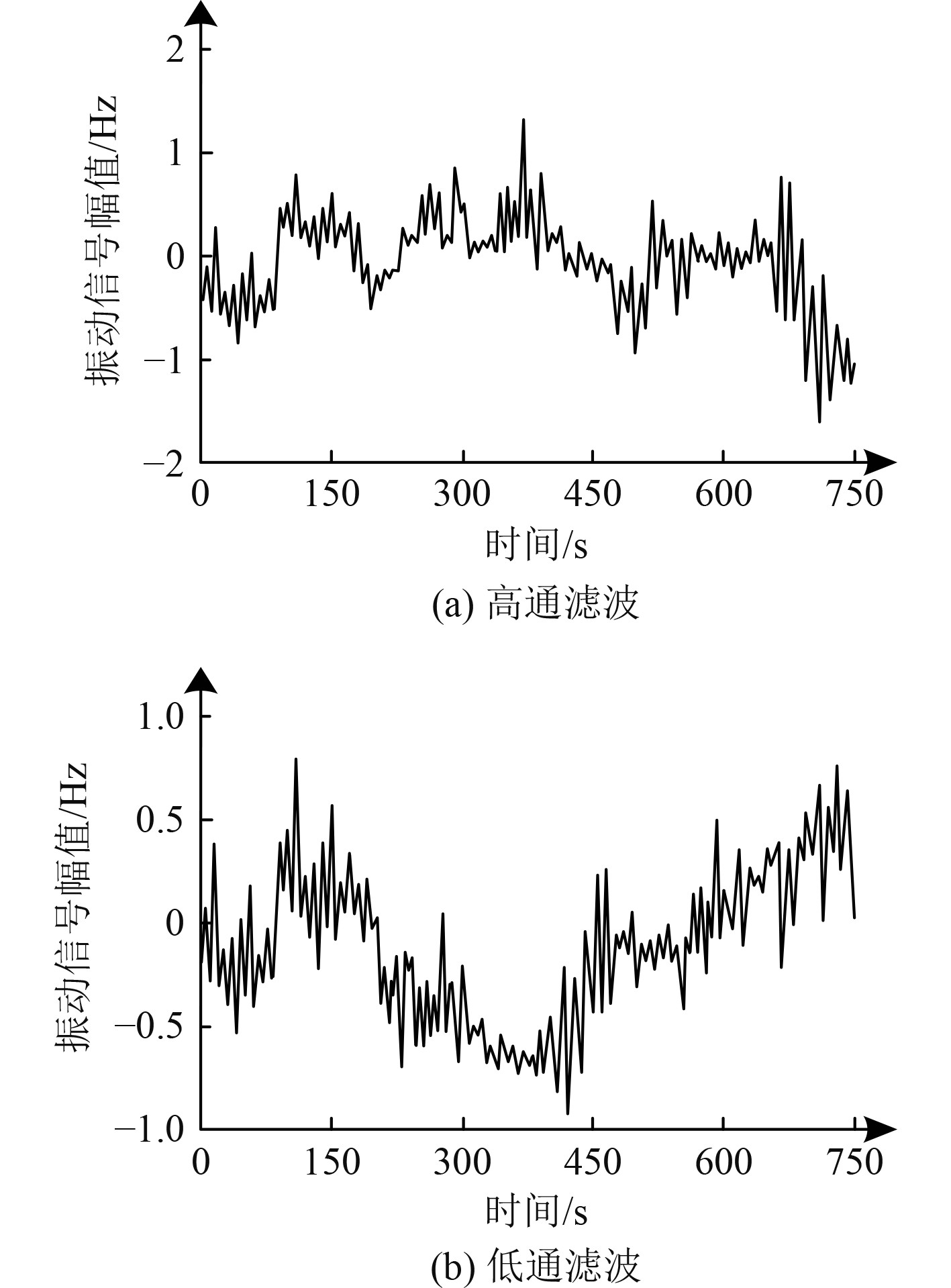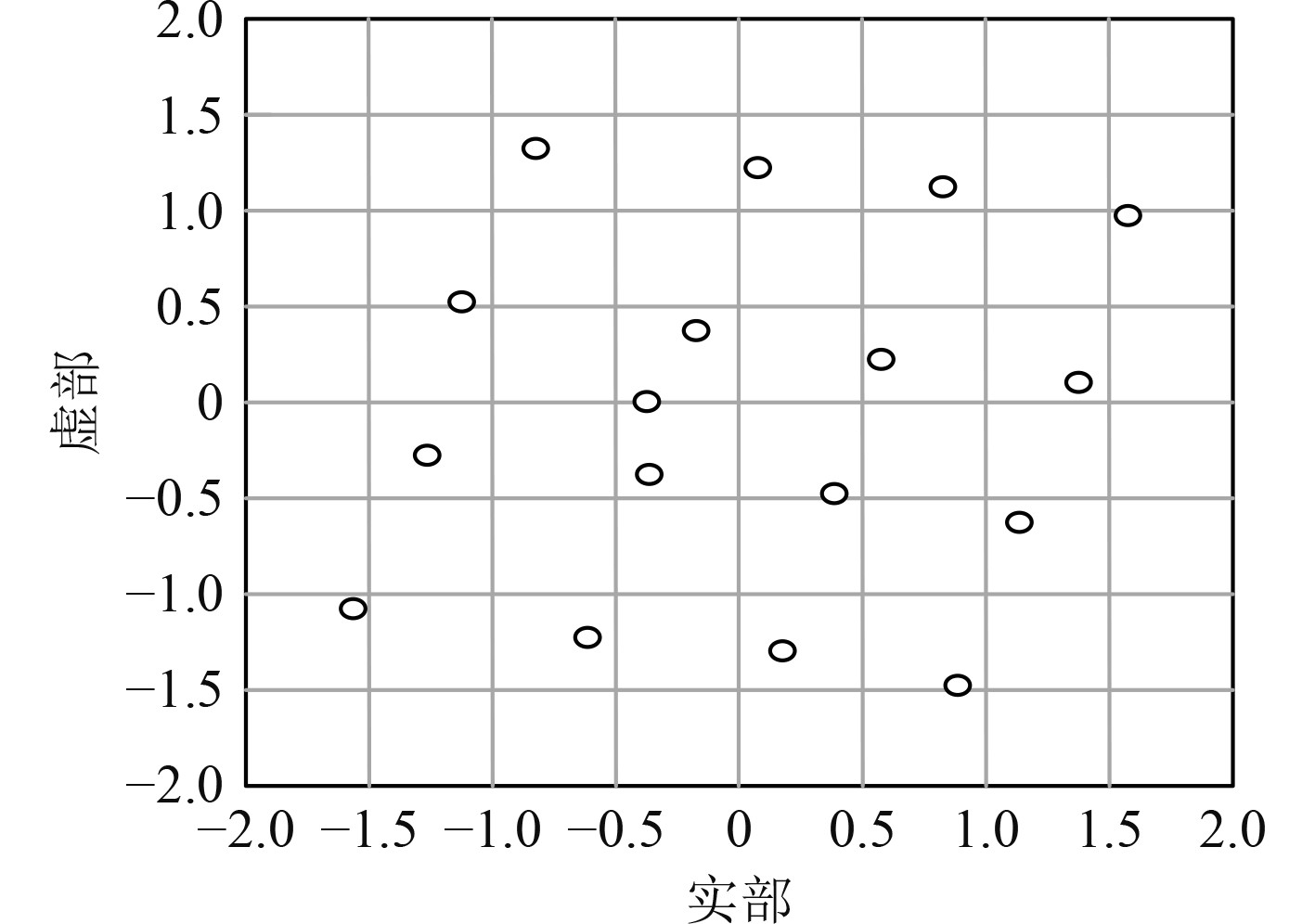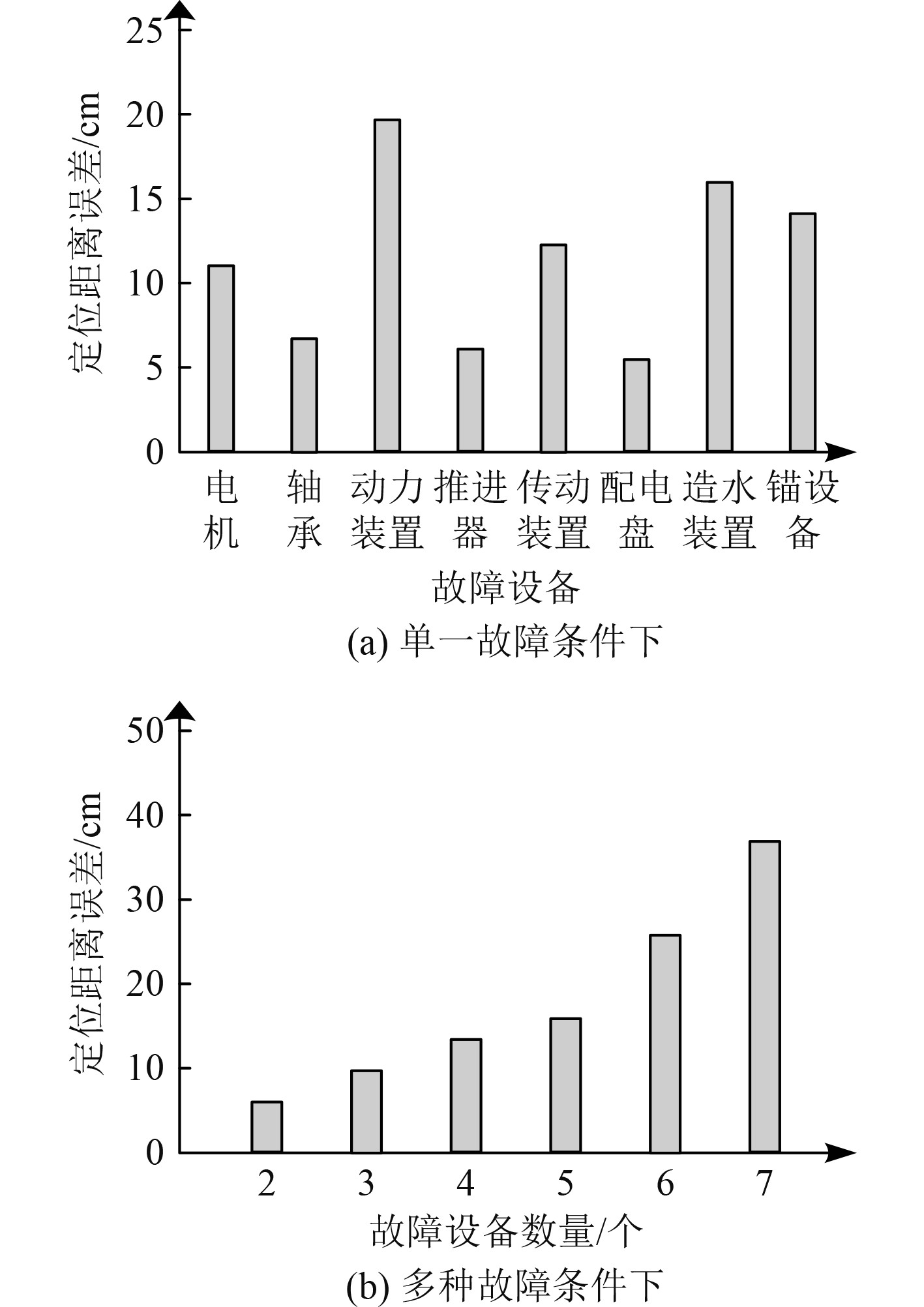﻿ 基于粒子群优化算法的舰船故障点智能定位研究
 舰船科学技术2022, Vol. 44Issue (10): 159-162    DOI: 10.3404/j.issn.1672-7649.2022.10.034PDF

Research on intelligent location of ship fault point based on particle swarm optimization algorithm
GAN Du-fen, CHEN Xiao-hai
The Computer Engineering College, Guilin University of Electronic Technology, Beihai 536000, China
Abstract: In order to ensure the safe navigation of ships, the intelligent location method of ship fault points based on particle swarm optimization algorithm is studied. Collect the vibration signal during the operation of ship mechanical equipment, and judge whether the operation condition of ship mechanical equipment is normal according to the vibration signal. According to the multi-resolution transformation of abnormal vibration signal based on wavelet packet theory, the orthogonal decomposition is implemented, and the feature vector is extracted on this basis; The eigenvectors are classified according to the correlation between self entropy and mutual entropy between abnormal vibration signals. Taking the vibration signal characteristics of ship equipment as the input of BP neural network model, the particle swarm optimization algorithm is used to globally search the optimal weight and threshold of BP neural network, and the parameter search efficiency is improved by determining the particle reverse fitness, so as to optimize the structure of BP neural network model and complete the purpose of ship fault identification and intelligent fault location. The experimental results show that the research method can accurately locate the fault point of mechanical equipment and accurately identify the fault of mechanical equipment under the condition of single fault or multiple faults.
Key words: particle swarm optimization     ship mechanical equipment     fault identification     fault location     vibration signal     BP neural network
0 引　言

1 基子粒子群优化算法的舰船故障点智能定位方法 1.1 舰船故障点智能定位方法架构设计

1.2 舰船机械设备异常振动信号特征提取

 $\left\{ \begin{gathered} {e_{2n + 1}}\left( x \right) = \sum\limits_i {F\left( i \right){e_n}\left( {2x - i} \right)} ，\hfill \\ {e_{2n}}\left( x \right) = \sum\limits_i {G\left( i \right){e_n}\left( {2x - i} \right)} 。\hfill \\ \end{gathered} \right.$ (1)

 $\begin{split} &{\lambda _f}\left( i \right) = \sum\limits_i {{\lambda _i}{F_{n - 2i}}}，\\ &{\lambda _G}\left( i \right) = \sum\limits_i {{\lambda _i}{G_{n - 2i}}} 。\end{split}$ (2)

 $z\left( x \right) = \sum\limits_i {\lambda _n^j{e_{2n}}} \left( {{2^{ - j - 1}}} \right) 。$ (3)

 $\left\| z \right\|_2^2 = \left( {\int {{{\left| {z\left( x \right)} \right|}^2}{\rm{d}}x} } \right) \times \theta 。$ (4)

 $\lambda = {\lambda _1} + {\lambda _2} + \ldots + {\lambda _m} 。$ (5)

 ${R_j} = \left( {\int {{{\left| {{\lambda _j}\left( t \right)} \right|}^2}dt} } \right) \times \theta = {\left| {{x_{jk}}} \right|^2} 。$ (6)

 $\sigma = \left\{ {{R_1},{R_2}, \cdots ,{R_m}} \right\} 。$ (7)

 $\left\{ \begin{gathered} \sigma = \frac{1}{R}\left\{ {{R_1},{R_2}, \cdots ,{R_m}} \right\} ，\hfill \\ R = \frac{{\left( {\displaystyle\sum\limits_{j = 1} {{{\left| {{R_j}} \right|}^2}} } \right)}}{2} 。\hfill \\ \end{gathered} \right.$ (8)

 ${\tau _i}\left( {{x_j}} \right) = - \sum\limits_{x \in X} {{p_j}\left( {x,{x_j}} \right)}。$ (9)

 ${\tau _{ij}}\left( {{x_i},{x_j}} \right) = {\tau _{ij}}\left( {{x_j},{x_i}} \right) - \sum\limits_{{x_j} \in {X_j}} {\sum\limits_{x \in {X_j}} {{p_{ij}}\left( {x,{x_i},{x_j}} \right)} } 。$ (10)

1.3 异常振动信号特征的船舶故障点识别与定位

1）输入层

 ${y_k} = \frac{{{x_k} - {x_{\min }}}}{{{x_{\max }} - {x_{\min }}}}。$ (11)

2）隐含层

 $h = \sqrt {\left( {m + n} \right)}。$ (12)

3）输出层

1.4 基于反向粒子群优化算法的神经网络优化

BP神经网络内权值与阈值的设定是影响最终网络性能的主要参数，因此可采用反向粒子群优化算法对这2个参数进行寻优，以此提升BP神经网络性能。采用粒子群优化算法优化BP神经网络过程中，先对一群随机粒子进行初始化处理，其中不同粒子均表示BP神经网络权值与阈值的可能解。各粒子的适应度取决于其位置坐标对应的目标函数值。经由追踪粒子自身所确定的最优解(定义为个体极值，用pb表示)和种群整体当前所确定的最优解(定义为全局极值，用gb表示)更新自身。

 $v_{ik}^{t + 1} = w \cdot v_{ik}^t + {b_1}\left( {{p_b}_{ik}^t - x_{ik}^t} \right) + {b_2}\left( {{g_b}_{ik}^t - x_{ik}^t} \right) ，$ (13)
 $x_{ik}^{t + 1} = x_{ik}^t + v_{ik}^{t + 1},i = 1,2, \cdots ,n;k = 1,2, \cdots ,K 。$ (14)

2 仿真实验 2.1 故障定位结果图 1 异常振动信号特征提取结果 Fig. 1 Feature extraction results of abnormal vibration signals图 2 故障点定位结果 Fig. 2 Fauit location results图 3 故障点定位精度 Fig. 3 Positioning accuracy of fault point
2.2 故障识别结果表 1 故障识别结果 Tab.1 Fault diagnosis results
3 结　论

  王瑞涵, 陈辉, 管聪. 基于机器学习的船舶机舱设备状态监测方法[J]. 中国舰船研究, 2021, 16(1): 158-167.  吴旭升, 杨刚, 孙盼, 等. 舰船电力电子设备测试性设计现状及技术分析[J]. 海军工程大学学报, 2021, 33(2): 14-18. DOI:10.7495/j.issn.1009-3486.2021.02.003  孙宇嫣, 蔡泽祥, 郭采珊, 等. 基于深度学习的智能变电站通信网络故障诊断与定位方法[J]. 电网技术, 2019, 43(12): 4306-4314.  周光宇, 马松龄. 基于机器学习与DGA的变压器故障诊断及定位研究[J]. 高压电器, 2020, 56(6): 262-268.  赵传宗, 王中杰, 穆景龙, 等. 基于小波变换和遗传算法的小电流接地故障定位技术研究[J]. 工业安全与环保, 2019, 45(1): 62-65.  郦阳, 王宝华. 继电保护系统故障的智能定位方法研究[J]. 电力系统保护与控制, 2022, 50(2): 69-76.  刘宇涛, 孙虎儿. 基于粒子群优化的CYCBD在滚动轴承故障特征提取的应用研究[J]. 机械传动, 2021, 45(2): 171-176.  张兰勇, 孟坤, 刘胜, 等. 基于改进双粒子群算法的舰船电力系统网络故障重构[J]. 电力系统保护与控制, 2201, 9,47(9): 90-96.  印波, 王锡淮, 肖健梅. 基于改进粒子群优化算法的船舶能量管理方案[J]. 中国舰船研究, 2020, 15(6): 37-45.